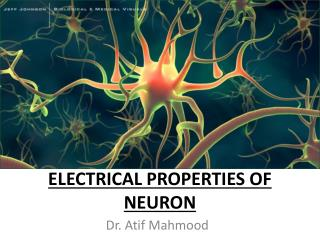DownloadDownload PresentationElectrical Properties of Neuron

# Electrical Properties of Neuron

Télécharger la présentation## Electrical Properties of Neuron

- - - - - - - - - - - - - - - - - - - - - - - - - - - E N D - - - - - - - - - - - - - - - - - - - - - - - - - - -
##### Presentation Transcript

1. Electrical Properties of Neuron Dr. Atif Mahmood

2. Learning Objectives At the end of the lecture, student should be able to:  • know the basic structure and function of neuron • understand its basic physical and electrical properties of neuron • to know the factors on which the resting membrane potential depends • to appreciate the mechanics of action potential initiation and measurements of action potentials. • Know the basics of patch-clamp technique

3. Two classes of cells • • Nerve cells (neurons) • is the basic structural and functional unit of nervous tissue • − For electrical signaling • − The human brain has 1011 neurons • • Glial cells • − Not for electrical signaling • − 10 to 50 times more glial cells than neurons • − Play an essential role in brain metabolism • −Support neurons • −Cover neurons with myelin • − Clean up debris

4. Neuron structure • cell body – contains nucleus & organelles • dendrite – conducts signals to cell body • axon – long fibers – specialized for electrical conduction

5. Structure of a typical neuron Dendrite Axon Terminal Node of Ranvier Cell Body Schwann Cell Axon Myelin Sheath Nucleus

6. Differences between neurons and other cells 1. Neurons have specialized extensions called dendrites and axons. Dendrites bringinformation to the cell body and axons takeinformation away from the cell body 2. Neurons communicate with each otherthrough an electrochemical process 3. Neurons contain some specialized structures(for example, synapses) and chemicals (forexample, neurotransmitters)

7. Morphology of Nerve cells (The Neuron)

8. Two processes extending from the cell body. (retinal cells, olfactory epithelium cells)

9. Galvani frog’s legs experiment

10. Outside Inside Ions and Ion Channels [Ca2+] [Cl-] [Na+] [K+] [K+] [Na+] [Ca2+] [Cl-] Cations: + Anions: - Channels are selective to particular ions. Passive vs. Active channels. Permeability is very high to K and Na, medium to Cl and very low to big anions. [K+]in=20 [K+]out [Na+]out=10 [Na+]in Main question: How is the membrane potential is related to [charges] in and out?

11. Outside Inside Equilibrium potential for one ion • Two competing forces: • Diffusion by concentration gradient. • Motion by voltage gradient. [K+] [K+] Diffusion Voltage difference

12. 1.3 Equilibrium potential for one ion Diffusion Flux: Jdif(x)= -D d[K+](x) / dx D, diffusion coefficient-> D=µkT/q µ, mobility k, Boltzmann constant q, ion charge [K+] x [K+] Voltage difference Flux: Jelec(x)= -µz [K+](x) dV(x) / dx µ, mobility z, ion valence, +/-1, +/-2, etc. Equilibrium happens when Jdif(x) + Jelec(x) = 0, which leads to the Nernst equation: E K+ = kT / zq ln [K+]out / [K+]in = -75,-90 mV E K+ is the potential necessary to maintain the concentration gradient [K+]out / [K+]in

13. 1.3 Equilibrium potential for one ion [K+] E K+ = kT / zq ln [K+]out / [K+]in = -75,-90 mV E Na+ = +55 mV E Ca2+ = +150 mV E Cl- = -60,-65mV x [K+] Na+ K+ K+ Na+ Vm = -70 mV E K+ < Vm < E Na+ Compensated by Na-K pump.

14. The Bulk Solution Remains Electroneutral

15. Ion Channel Selectivity and Ionic Concentration Gradient Result in an Electromotive Force

16. The Lipid Bilayer Acts Like a Capacitor • Cell membrane: 2-3 nm thick and is impermeable to most charged molecules and so acts as a capacitor by separating the charges lying on either side of the membrane. • NB Capacitors, store charge across an insulating medium. Don’t allow current to flow across, but charge can be redistributed on each side leading to current flow.

17. The Lipid Bilayer Acts Like a Capacitor The voltage (Vm)across a capacitor is proportional to the charge (Q) stored on the capacitor: + + + + Vm = Q/C - - - - ∆Vm = ∆Q/C ∆Q must change before ∆Vm can change

18. Capacitance is Proportional to Membrane Area + - + + - - + + + - - - - + + + + - - Vm = Q/C - - + + - + - + + + - - - - - - - + + + + - - - + + + - +

19. + + + + Electrical Signaling in the Nervous System isCaused by theOpening or Closing of Ion Channels - + - - + + - + - + - + + - - + - The Resultant Flow of Charge into the Cell Drives the Membrane Potential Away From its Resting Value

20. Equilibrium and reversal potentials Equilibriumpotentials (The Nernstequation applies when the channels allow only one type of ion to pass through them) Some channels are not so selective, and in this case the potential E is estimated by the Goldman equation Reversal potentials takes a value intermediate between the equilibrium potentials of the individual ion types that it conducts